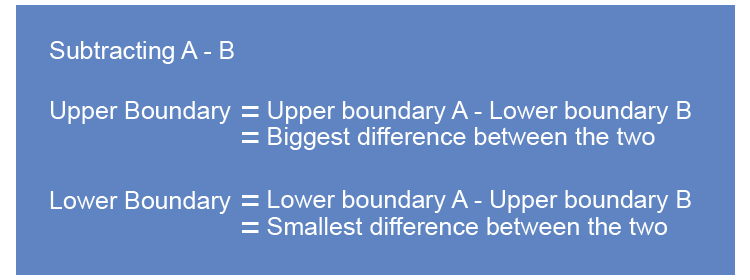# Upper and lower boundary subtraction

If you are looking to subtract one quantity from another and you need to know the possible limits upper boundary and lower boundary use logic.NOTE:

1. Upper boundary = Upper boundary A- Lower boundary B

If you have the largest possible amount and subtract the smallest possible amount this will provide you with the biggest quantity difference possible.

Upper boundary = Big - Small = Largest difference

2. Lower boundary = Lower boundary A- Upper boundary B

If you have the smallest possible amount and subtract the largest possible amount this will provide you with the smallest difference between the two.

Lower boundary = Small - Big = Smallest difference

Example 1

Two neighbours grew a sunflower in their gardens. Gail's sunflower grew to 2.3metres and Davina's grew to 1.8metres. You found out that the measurement had been made to two significant figures.

What are the maximum and minimum differences between Gail's taller plant and Davina's?

This question is a subtraction question but the first thing you must do is find the maximum and minimum values of each number.

The upper and lower boundary of 2.3metres is

2.3m to 2 significant figures.

2.ul3 underline the last digit.

2.ul3\0 look next door

0      5 or more raises the score. So 2.25 would raise to 2.3.

0       four or less just ignore 2.3499 it would ignore and lower to 2.3.

This is simplified to 2.35

So the upper and lower boundary of 2.3metres is 2.35m and 2.25m.

The upper and lower boundary of 1.8metres is

1.8m to 2 significant figures.

1.ul8 underline the last digit.

1.ul8\0 look next door

0      5 or more raises the score. So 1.75 would raise to 1.8.

0       four or less just ignore 1.8499 it would ignore and lower to 1.8.

This is simplified to 1.85

So the upper and lower boundary of 1.8metres is 1.85m and 1.75m.

Summary

Upper and lower boundary of 2.3metres is 2.35m and 2.25m.

Upper and lower boundary of 1.8metres is 1.85m and 1.75m.

The largest difference between the two heights would be the biggest possible difference between their boundaries.

 Upper boundary = Upper boundary A - Lower boundary B Upper boundary = 2.35 - 1.75 =0.6m Big Small

The smallest difference between the two heights would be the smallest possible difference between their boundaries.

 Lower boundary = Lower boundary A - Upper boundary B Lower boundary = 2.25 - 1.85 =0.4m Small Big

Answer: The maximum and minimum differences between the two plants are 0.6m and 0.4m.

Example 2

Sheila earns $1800 per month and Mary earns$1200 per month to the nearest $100. What are the minimum and maximum differences in their salary per month? This question is a subtraction question but the first thing you must do is find the maximum and minimum values of each number. The upper and lower boundary of $1800 is

$1800 to the nearest $100.

1ul8\00 underline the last digit.

1ul8\00 look next door

0      5 or more raises the score. So 1750 would raise to 1800.

0       four or less just ignore 1849.9dot9 it would ignore and lower to 1800.

This is simplified to 1850

So the upper and lower boundary of $1800 is $1850 and $1750. The upper and lower boundary of $1200 is

$1200 to the nearest $100.

1ul2\00 underline the last digit.

1ul2\00 look next door

0      5 or more raises the score. So 1150 would raise to 1200.

0       four or less just ignore 1249.9dot9 it would ignore and lower to 1200.

This is simplified to 1250

So the upper and lower boundary of $1200 is $1250 and $1150. Summary Upper and lower boundary of $1800 is $1850 and $1750.

Upper and lower boundary of $1200 is $1250 and $1150. The largest difference between these two monetary sums would be the biggest possible difference between their boundaries.  Upper boundary = Upper boundary A - Lower boundary B Upper boundary = $1850 - $1150 =$700 Big Small

The smallest difference between these two monetary sums would be the smallest possible difference between their boundaries.

 Lower boundary = Lower boundary A - Upper boundary B Lower boundary = $1750 - $1250 =$500 Small Big Answer: The maximum and minimum differences between their two salaries is $700 and \$500.